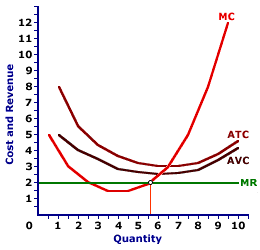Friday  December 1, 2023
 AmosWEB means Economics with a Touch of Whimsy!NOMINAL GDP: The total market value, measured in current prices, of all goods and services produced within the political boundaries of an economy during a given period of time, usually one year. The key is that nominal gross domestic product is measured in current, or actual prices; the prices buyers actually pay for goods and services purchased. Nominal gross domestic product is also termed current gross domestic product.SHUTDOWN RULE:

A rule stating that a firm minimizes economic loss by producing no output in the short run if price is less than average variable cost. This is one of three short-run production alternatives facing a firm. The other two are profit maximization (if price exceeds average total cost) and loss minimization (if price is less than average total cost but greater than average variable cost).
Production Alternatives
Price and CostResult
P > ATCProfit Maximization
ATC > P > AVCLoss Minimization
P < AVCShutdown
The shutdown rule applies to a firm that is incurring a short-run economic loss that exceeds total fixed cost. This occurs if the price received is less than average variable cost. It is not an absolute rule so much as it is an alternative that any profit maximizing firm is inclined to pursue given production cost and market conditions.

Shutting down is one of three short-run production alternatives facing a firm. All three are displayed in the table to the right. The other two are profit maximization and loss minimization.

• With profit maximization, price exceeds average total cost at the quantity that equates marginal revenue and marginal cost. In this case, the firm generates an economic profit.

• With loss minimization, price exceeds average variable cost but is less than average total cost at the quantity that equates marginal revenue and marginal cost. In this case, the firm incurs a smaller loss by producing some output than by not producing any output.
In the short run, a firm incurs total fixed cost whether or not it produces any output. As such, if the market price falls below average total cost, it must decide if the economic loss from producing the quantity of output that equates marginal revenue and marginal cost is more or less than the economic loss incurred with shutting down production in the short run (which is equal to total fixed cost).

Shutting DownThe key criterion for this decision is price relative to average variable cost.

• If price is less than average variable cost, a firm does not receive enough revenue to pay variable cost let alone any part of fixed cost. As such, the economic loss of operating is GREATER than total fixed cost. A firm is better off shutting down production in the short run, producing zero output, and awaiting a higher price.

• If price is greater than average variable cost, a firm receives sufficient revenue to pay ALL variable cost plus some fixed cost. As such, the economic loss is LESS than total fixed cost. A firm is better off producing the quantity that equates marginal revenue and marginal cost than producing no output, receiving no revenue and incurring a loss equal to total fixed cost.
The exhibit here illustrates the shutdown situation that exists for a hypothetical perfectly competitive firm, Phil the zucchini-growing gardener. The going market price (marginal revenue) of \$2 per pound of zucchinis received by Phil, intersects the marginal cost curve at less than the average variable cost curve.

Because price falls short of average variable cost, Phil incurs a loss. However, because the price is less than average variable cost, Phil incurs a smaller loss by shutting down production and incurring the loss of total fixed cost, than by producing any output.

 <= SHORTAGE SIGNALLING =>Recommended Citation:

SHUTDOWN RULE, AmosWEB Encyclonomic WEB*pedia, http://www.AmosWEB.com, AmosWEB LLC, 2000-2023. [Accessed: December 1, 2023].

Check Out These Related Terms...

Or For A Little Background...

And For Further Study...
Search Again?BEIGE MUNDORTLE[What's This?] Today, you are likely to spend a great deal of time touring the new suburban shopping complex seeking to buy either a replacement nozzle for your shower or a decorative windchime with plastic . Be on the lookout for mail order catalogs with hidden messages.Your Complete ScopeThe word "fiscal" is derived from a Latin word meaning "moneybag.""Sometimes our light goes out, but is blown into flame by another human being. Each of us owes deepest thanks to those who have rekindled this light. "-- Albert Schweitzer, missionary physicianNTBNon-Tariff BarrierA PEDestrian's Guide Xtra CreditTell us what you think about AmosWEB. Like what you see? Have suggestions for improvements? Let us know. Click the User Feedback link.| | | | | | | | | | |
| | | |

Thanks for visiting AmosWEB